## 20071031

### Physics quiz question: impulse-driven objects

Physics 5A Quiz 4, Fall Semester 2007
Cuesta College, San Luis Obispo, CA

Cf. Giambattista/Richardson/Richardson, Physics, 1/e, Conceptual Question 7.2(b)

[Version 1]

[3.0 points.] An impulse of 50 N*s is applied to each of two objects of different masses that are initially at rest. Which object has the greatest velocity change?
(A) The less massive object.
(B) The more massive object.
(C) (Both objects have the same velocity change.)
(D) (Not enough information is given to determine this.)

Impulse = delta(p) = m*(v_f - v_i). With v_i = 0 for both objects, v_f will be greater for the less massive object, and v_f will be smaller for the more massive object.

Student responses
Sections 0906, 0907
(A) : 15 students
(B) : 4 students
(C) : 2 students
(D) : 0 students

[Version 2]

[3.0 points.] Two objects of different masses are initially at rest, and then undergo the same velocity change. Which object experienced the greater impulse?
(A) The less massive object.
(B) The more massive object.
(C) (Both objects experienced the same impulse.)
(D) (Not enough information is given to determine this.)

Impulse = delta(p) = m*(v_f - v_i). With v_i = 0 for both objects, and the same v_f as well, a greater impulse would be exerted on the more massive object, and a smaller impulse would be exerted on the less massive object.

Student responses
Sections 0906, 0907
(A) : 5 students
(B) : 13 students
(C) : 1 student
(D) : 0 students

## 20071030

### Physics clicker questions: torque sequence

Physics 5A, Fall Semester 2007
Cuesta College, San Luis Obispo, CA

Cf. Giambattista/Richardson/Richardson, Physics, 1/e, Multiple-Choice Question 8.6

Students were asked the following clicker questions (Classroom Performance System, einstruction.com) near the end of their learning cycle: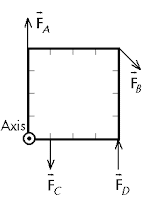[0.6 participation points.] Which one of the forces in the figure produces the largest magnitude torque about the rotation axis indicated? (All forces have the same magnitude.)
(A) F_A.
(B) F_B.
(C) F_C.
(D) F_D.
(E) (More than one of the forces listed above has the largest magnitude torque.)
(F) (I'm lost, and don't know how to answer this.)

Sections 0906, 0907
(A) : 2 students
(B) : 21 students
(C) : 7 students
(D) : 5 students
(E) : 4 students
(F) : 0 students

Force A has zero torque, as it is parallel to its lever arm. All of the remaining forces are perpendicular to their lever arms. Force C and force D provide some torque, but force B has the greatest torque, due to having the longest lever arm.

[0.6 participation points.] How many of the forces in the figure above produces a positive torque about the rotation axis indicated? _____.

Sections 0906, 0907
0 : 1 student
1 : 29 students
2 : 8 students
3 : 1 student
4 : 0 students

Force B and force C would turn the square clockwise, and thus exert negative torques. Force D would turn the square counterclockwise, and thus would exert a positive torque.

## 20071029

### Physics clicker questions: payload and toy car sequence

Physics 5A, Fall Semester 2007
Cuesta College, San Luis Obispo, CA

Cf. Giambattista/Richardson/Richardson, Physics, 1/e, Problem 7.40

Students were asked the following clicker questions (Classroom Performance System, einstruction.com) near the end of their learning cycle:

Object 1 = toy car
i = toy car moving to the right, before payload is dropped down onto it
f = toy car after payload has been dropped on it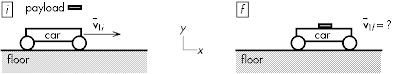[0.6 participation points.] What is the final speed of the toy car? (Floor is frictionless.)
(A) Slower than its initial speed.
(B) Same as its initial speed.
(C) Faster than its initial speed.
(D) (Not enough information is given to determine this.)
(E) (I'm lost, and don't know how to answer this.)

Sections 0906, 0907
(A) : 27 students
(B) : 5 students
(C) : 4 students
(D) : 0 students
(E) : 0 students

Since there are no external horizontal impulses, momentum is conserved, such that v_12,f = (m_1*v_1,i)/(m_1 + m2).

Object 1 = toy car
i = toy car moving to the right, carrying payload
f = toy car after payload has been dropped (down into hole in floor)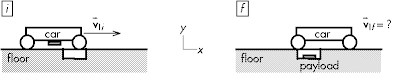[0.6 participation points.] What is the final speed of the toy car? (Floor is frictionless.)
(A) Slower than its initial speed.
(B) Same as its initial speed.
(C) Faster than its initial speed.
(D) (Not enough information is given to determine this.)
(E) (I'm lost, and don't know how to answer this.)

Sections 0906, 0907
(A) : 4 students
(B) : 19 students
(C) : 13 students
(D) : 0 students
(E) : 0 students

Although not classifiable as a collision, after the payload separates from the toy car (but before it hits the ground) it will have the same velocity as the car, as there are no external horizontal impulses.

Many students will misapply the definition of momentum to a conservation of momentum situation.

p = m*v.
• For the payload dropped down onto the toy car: p = m*v, where m increases, thus v decreases. (Seems correct?)
• For the payload dropped down from the toy car: p = m*v, where m decreases, thus v increases. (No!)
More correctly, conservation of momentum should be applied properly as:

0 = delta(p_1) + delta(p_2).
• For the payload dropped down onto the toy car, the payload gains horizontal momentum, thus the car loses horizontal momentum.
• For the payload dropped down from the toy car, the payload retains the same horizontal momentum, thus the car's horizontal momentum remains the same!

## 20071026

### Physics clicker questions: bullet into block sequence

Physics 5A, Fall Semester 2007
Cuesta College, San Luis Obispo, CA

Cf. Giambattista/Richardson/Richardson, Physics, 1/e, Problem 7.44

Students were asked the following clicker questions (Classroom Performance System, einstruction.com) near the end of their learning cycle:

Object 1 = 0.020 kg bullet
Object 2 = 2.00 kg block
i = bullet before hitting block, block still stationary
f = just after bullet embedded into block, but before any sliding actually takes place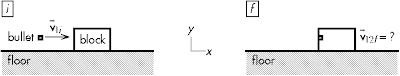[0.6 participation points.] What is conserved for the process i→f?
(A) Momentum.
(B) Kinetic energy.
(C) Energy, with non-conservative work being done.
(D) (More than one of the above choices apply.)
(E) (Nothing is conserved.)
(F) (I'm lost, and don't know how to answer this.)

Sections 0906, 0907
(A) : 25 students
(B) : 3 students
(C) : 1 students
(D) : 7 students
(E) : 0 students
(F) : 0 students

Since there are no external horizontal impulses, momentum is conserved, such that v_12,f = (m_1*v_1,i)/(m_1 + m2). However, since there is deformation with no rebound and separation in this perfectly inelastic collision, kinetic energy is not conserved.

f = just after bullet embedded into block, but before any sliding actually takes place
ff = after block (with bullet inside) comes to a rest because of kinetic friction[0.6 participation points.] What is conserved for the process f→ff?
(A) Momentum.
(B) Kinetic energy.
(C) Energy, with non-conservative work being done.
(D) (More than one of the above choices apply.)
(E) (Nothing is conserved.)
(F) (I'm lost, and don't know how to answer this.)

Sections 0906, 0907
(A) : 4 students
(B) : 9 students
(C) : 8 students
(D) : 8 students
(E) : 5 students
(F) : 1 students

Friction causes a horizontal impulse on the block (with embedded bullet), and thus momentum is not conserved. Friction also does work on the block/bullet, thus kinetic energy is not conserved, but energy is conserved if the non-conservative work done by friction is accounted for.

W_nc = delta(K_12),
-mu_k*(m_1 + m_2)*g*delta_x = (1/2)*(m_1 + m_2)*((v_12,f)^2 - 0).

## 20071025

### Physics clicker questions: kinetic energy-momentum sequence

Physics 5A, Fall Semester 2007
Cuesta College, San Luis Obispo, CA

Cf. Giambattista/Richardson/Richardson, Physics, 1/e, Problem 7.7

Students were asked the following clicker questions (Classroom Performance System, einstruction.com) near the beginning of their learning cycle:

Mass of (bowling?) ball = 5.0 kg

[0.6 participation points.] The ball is initially at rest, and then you push it such that it has a final speed of 3.0 m/s in the +x direction. What was the change in kinetic energy of the ball?
(A) –23 J.
(B) –15 J.
(C) 0 J.
(D) +15 J.
(E) +23 J.
(F) (I'm lost, and don't know how to answer this.)

Sections 0906, 0907
(A) : 1 student
(B) : 1 student
(C) : 1 student
(D) : 9 students
(E) : 22 students

[0.6 participation points.] What was the change in momentum of the ball?
(A) –23 kg*m/s.
(B) –15 kg*m/s.
(C) 0 kg*m/s.
(D) +15 kg*m/s.
(E) +23 kg*m/s.
(F) (I'm lost, and don't know how to answer this.)

Sections 0906, 0907
(A) : 0 students
(B) : 0 students
(C) : 0 students
(D) : 32 students
(E) : 2 students
(F) : 0 students

So far, so good--students seem to understand how to calculate changes in kinetic energy, and changes in momentum, especially when the initial velocity is zero.

Mass of (bowling) ball = 5.0 kg

[0.6 participation points.] The ball is moving with a speed of 3.0 m/s in the +x direction, and then hits a wall and bounces back with the same speed in the –x direction. What was the change in kinetic energy of the ball?
(A) –23 J.
(B) –15 J.
(C) 0 J.
(D) +15 J.
(E) +23 J.
(F) (I'm lost, and don't know how to answer this.)

Sections 0906, 0907
(A) : 1 student
(B) : 2 students
(C) : 25 students
(D) : 0 students
(E) : 5 students
(F) : 0 students

The final and initial speed is the same, so there is no change in kinetic energy.

[0.6 participation points.] What was the change in momentum of the ball?
(A) –23 kg*m/s.
(B) –15 kg*m/s.
(C) 0 kg*m/s.
(D) +15 kg*m/s.
(E) +23 kg*m/s.
(F) (I'm lost, and don't know how to answer this.)

Sections 0906, 0907
(A) : 27 student
(B) : 2 students
(C) : 5 students
(D) : 0 students
(E) : 0 students
(F) : 0 students

The final velocity is negative, and the initial velocity is positive, such that the change in momentum (the impulse) points in the -x direction. (Note that a negative change in kinetic energy corresponds to a decrease in speed; a negative change in momentum corresponds to the -x direction.)

## 20071024

### Astronomy midterm question: cooler Uranus

Astronomy 10 Midterm 2, Fall Semester 2007
Cuesta College, San Luis Obispo, CA

Astronomy 10 learning goal Q2.2

[15 points.] Discuss the evidence that supports the theory that the interior of Uranus is cooler than the interior of Neptune.

• p = 15/15: Correct. The lack of belt-zone and cyclonic weather patterns on Uranus, which are generated by convection from core heat is evidence that the interior of Uranus is cooler than the interior of Neptune. The tilted axis of Uranus is evidence that may explain how a large impact may have caused a "Cooper Cooler(TM) Effect" (stirred fluids cool off faster than static fluids).
• r = 12/15:
Nearly correct (explanation weak, unclear or only nearly complete); includes extraneous/tangential information; or has minor errors. Points out tilted axis of Uranus, but argues that sideways spin results in cooler interior temperatures for Uranus, and not how the tilted axis may have been the result of a large impact that would have disrupted and stirred its interior.
• t = 9/15:
Contains right ideas, but discussion is unclear/incomplete or contains major errors. Explains how the "Cooper Cooler(TM) Effect" works, but does not discuss evidence that this may have occurred for Uranus.
• v = 6/15:
Limited relevant discussion of supporting evidence of at least some merit, but in an inconsistent or unclear manner. May appeal to the "Turkey/Cornish Hen Effect" (smaller objects cool off faster than larger objects).
• x = 3/15:
Implementation/application of ideas, but credit given for effort rather than merit.
• y = 1.5/15:
Irrelevant discussion/effectively blank.
• z = 0/15:
Blank.
Section 0135
p: 15 students
r: 6 students
t: 5 students
v: 9 students
x: 3 students
y: 0 student
z: 0 students

Section 1073
p: 30 students
r: 3 students
t: 6 students
v: 5 students
x: 2 students
y: 0 students
z: 1 student

A sample of a "p" response (from student 5150) is shown below: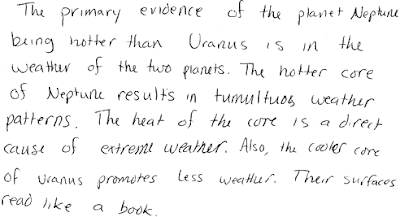## 20071023

### Astronomy midterm question: runaway greenhouse or refrigerator

Astronomy 10 Midterm 2, Fall Semester 2007
Cuesta College, San Luis Obispo, CA

Astronomy 10 learning goal Q5.5

[Version 1]
[15 points.] If volcanic activity drastically increased on the Earth, discuss how it would either become a runaway refrigerator (like Mars), or a runaway greenhouse (like Venus). Explain using properties of the geological/atmospheric cycles of planets.

• p = 15/15: Correct. Increasing volcanic activity means more carbon dioxide released back from the mantle into the atmosphere, increasing the greenhouse effect and causing cooler temperatures. The runaway greenhouse effect is when it is hot enough for the oceans to evaporate, such that water vapor (also a greenhouse gas) is now prevalent in the atmosphere, and thus even hotter temperatures will take place.
• r = 12/15:
Nearly correct (explanation weak, unclear or only nearly complete); includes extraneous/tangential information; or has minor errors. Explains how Earth's greenhouse effect will get stronger and hotter temperatures would result, but not how the evaporation of oceans and prevalence of water vapor will accelerate this effect.
• t = 9/15:
Contains right ideas, but discussion is unclear/incomplete or contains major errors. Understands how carbon dioxide produces a greenhouse effect, and how an increase in volcanic activity would generate higher temperatures.
• v = 6/15:
Limited relevant discussion of supporting evidence of at least some merit, but in an inconsistent or unclear manner.
• x = 3/15:
Implementation/application of ideas, but credit given for effort rather than merit.
• y = 1.5/15:
Irrelevant discussion/effectively blank.
• z = 0/15:
Blank.
Section 0135
p: 7 students
r: 8 students
t: 3 students
v: 13 students
x: 6 students
y: 1 student
z: 0 students

A sample of a "p" response (from student 2001) is shown below: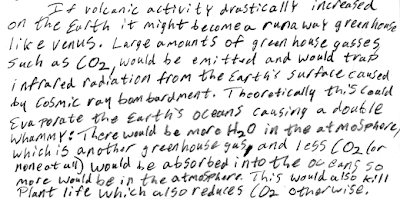[Version 2]
[15 points.] If volcanic activity drastically increased on the Earth, discuss how it would either become a runaway refrigerator (like Mars), or a runaway greenhouse (like Venus). Explain using properties of the geological/atmospheric cycles of planets.

• p = 15/15: Correct. Tectonic plate motion recycles sedimentary rock of ocean floors (which contains carbon dioxide from the atmosphere) down into the mantle. Decreasing volcanic activity means less carbon dioxide released back from the mantle into the atmosphere, decreasing the greenhouse effect and causing cooler temperatures. The runaway refrigerator effect is when it is cold enough for the oceans to freeze, such that water vapor (also a greenhouse gas) is no longer present in the atmosphere, and thus even colder temperatures will take place.
• r = 12/15:
Nearly correct (explanation weak, unclear or only nearly complete); includes extraneous/tangential information; or has minor errors. Explains how Earth's greenhouse effect will get weaker and cooler temperatures would result, but not how the freezing oceans and lack of water vapor will accelerate this effect.
• t = 9/15:
Contains right ideas, but discussion is unclear/incomplete or contains major errors. Discusses how no new carbon dioxide put into the atmosphere by volcanos would result in lower temperatures, without mentioning that the process that removes carbon dioxide from the atmosphere is still continuing.
• v = 6/15:
Limited relevant discussion of supporting evidence of at least some merit, but in an inconsistent or unclear manner. May discuss how lack of volcanos and continuing tectonic plate motion will result in a runaway greenhouse effect.
• x = 3/15:
Implementation/application of ideas, but credit given for effort rather than merit.
• y = 1.5/15:
Irrelevant discussion/effectively blank.
• z = 0/15:
Blank.
Section 1073
p: 1 student
r: 13 students
t: 13 students
v: 15 students
x: 5 students
y: 0 students
z: 0 students

A sample of a "p" response (from student 7823) is shown below:## 20071022

### Physics clicker question: airbag effect

Physics 5A, Fall Semester 2007
Cuesta College, San Luis Obispo, CA

Cf. Giambattista/Richardson/Richardson, Physics, 1/e, Example 7.3

Students were asked the following clicker question (Classroom Performance System, einstruction.com) in the middle of their learning cycle:

[0.6 participation points.] An airbag (in conjunction with a seatbelt) will lessen the injuries caused by a car accident. How does the airbag do this, compared to wearing a seatbelt only?
(A) By decreasing the change in momentum.
(B) By decreasing the amount of impulse.
(C) By increasing the time duration of impact.
(D) (More than one of the above choices apply.)
(E) (I'm lost, and don't know how to answer this.)

Sections 0906, 0907
(A) : 5 students
(B) : 5 students
(C) : 11 students
(D) : 14 students
(E) : 0 students

Assuming that the same type of accident is involved for the same mass occupant, the momentum change (m*(v_f - v_i) will be the same, as will be the impulse on the driver. The time duration (delta_t) for the impulse (F*delta_t) to be applied will be longer with the airbag, thus the force applied on the occupant will be lessened.

## 20071019

### Airbags make babies cry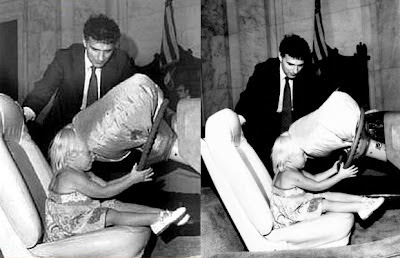L: Ralph Nader and Toddler, (© UPI/Bettman)
achievement.org
July 5, 1977

R: Ralph Nader and Toddler, unknown photographer
scienceservingsociety.com
July 5, 1977

Sure, airbags save lives, but do airbags make babies cry?

Two photos from the same event. Ralph Nader coaxing little Betsy into the airbag demonstrator, placing his finger over the airbag detonator.

"Watch out for that tree, Betsy! Watch out! Don't you see it? Betsy, what are you doing?!? BETSY..."

<INFLATE>Poof.</INFLATE>

A good 30 seconds passes before Betsy begins bawling her eyes out.

Well, that's the way it might have happened.

## 20071018

### American crash-test dummiesBizarro, by Dan Piraro
www.bizarro.com
March 30, 2007

Considering the same velocity change for a typical accident (v_f - v_i), a larger mass will require a greater impulse to come to a complete stop.

## 20071017

### Making diamondsBizarro, by Dan Piraro
www.bizarro.com
June 19, 2007

Astronomy 10 learning goals Q4.4, Q6.1

Diamond is tetrahedrally configured carbon, which can be made after subjected to immense pressures and high temperatures, such as in the mantle of the Earth, in the cores of jovian planets.

## 20071015

### Physics midterm question: thrown upwards vs. downwards balls

Physics 5A Midterm 1, fall semester 2007
Cuesta College, San Luis Obispo, CA

Cf. Giambattista/Richardson/Richardson, Physics, 1/e, Conceptual Question 3.6

[10 points.] You are standing on a balcony overlooking the beach. You throw and release a ball straight up into the air with an initial speed, and throw and release an identical ball straight down with the same initial speed. Neglect air resistance. Why would they have the same speed when they hit the ground (at different times)? Explain your reasoning using the properties of constant acceleration motion.

• p = 10/10: Correct.
Argues that the ball that was tossed straight up with an initial speed will come back down past its starting point with the same speed, and thus will fall downwards in the same manner from this point onwards as the ball thrown downwards with the same initial speed. Quantitatively this comes from vfy2 - viy2 = 2·ay·∆y because of the square of a positive or negative viy will result in the same vfy, but a thorough qualitative argument is sufficient.
• r = 8/10:
As (p), but argument indirectly, weakly, or only by definition supports the statement to be proven, or has minor inconsistencies or loopholes.
• t = 6/10:
Nearly correct, but argument has conceptual errors, or is incomplete. Some attempt at incorporating impulse and momentum in discussion. May use a graph, or equations, or argues qualtitatively that the ball that is thrown upwards will start moving downwards from a higher height with zero velocity, and thus will speed up over a greater downward distance to match the final speed of the downwards thrown ball.
• v = 4/10:
Limited relevant discussion of supporting evidence of at least some merit, but in an inconsistent or unclear manner. Argument based on same acceleration, and thus same final velocity, without appealing to how results from vfy2 - viy2 = 2·ay·∆y apply, or from the qualitative explanation given in response (p).
• x = 2/10:
Implementation/application of ideas, but credit given for effort rather than merit. Interprets final velocities of balls when they hit the ground as being zero (which would be true after they have hit the ground).
• y = 1/10:
Irrelevant discussion/effectively blank.
• z = 0/10:
Blank.

p: 19 students
r: 1 student
t: 17 students
v: 6 students
x: 0 students
y: 0 students
z: 0 students

A sample of a "p" response (from student 0036) is shown below: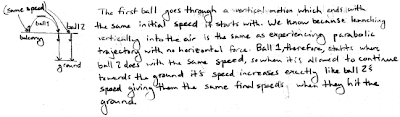## 20071012

### Physics midterm problem: diagonally thrown-downwards beanbag

Physics 5A Midterm 1, fall semester 2007
Cuesta College, San Luis Obispo, CA

Cf. Giambattista/Richardson/Richardson, Physics, 1/e, Problem 3.42(c)

[20 points.] A beanbag is thrown with a velocity of 15 m/s at 45° below the horizontal from a window a height 30 m above the ground. At what horizontal distance from the point directly below the window will the beanbag hit the level ground? Neglect air resistance. Show your work and explain your reasoning.

• p = 20/20: Correct.
Breaks the initial velocity vector in separate x- and y-components. Note that ax = 0, and ay = -9.80 m/s2. May either use quadratic equation from ∆y = viy·∆t + (1/2)·ay·(∆t)2, to solve for time; or vfy2 - viy2 = 2·ay·∆y and then plugging in vfy into vfy = viy + ay·∆t to find time. Once time is known, it can then be used to find the horizontal displacement ∆x when the beanbag hits the ground.
• r = 16/20:
Nearly correct, but includes minor math errors. Otherwise carries out systematic decomposition into x and y-components, to kinematic equations, to reduction/substitution using algebra approach.
• t = 12/20:
Nearly correct, but approach has conceptual errors, and/or major/compounded math errors.
• v = 8/20:
Implementation of right ideas, but in an inconsistent, incomplete, or unorganized manner. Still has a methodical approach based on the kinematic equations of motion.
• x = 4/20:
Implementation of ideas, but credit given for effort rather than merit. May estimate distance from by appealing to trigonometry and straight-line travel.
• y = 2/20:
Irrelevant discussion/effectively blank.
• z = 0/20:
Blank.
p: 7 students
r: 6 students
t: 5 students
v: 19 students
x: 6 students
y: 0 students
z: 0 students

A sample of a "p" response (from student 2325) using the quadratic formula to first determine the time for the beanbag to hit the ground: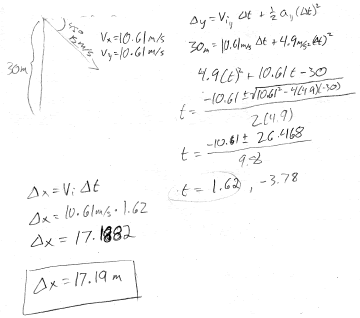A sample of a "p" response (from student 3153) instead solving for the final vertical velocity of the beanbag in order to solve for the time for the beanbag to hit the ground: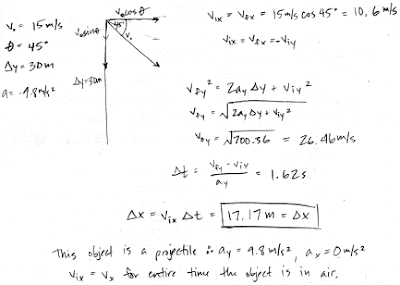## 20071011

### Physics clicker question: circular motion free-body diagrams

Physics 5A, Fall Semester 2007
Cuesta College, San Luis Obispo, CA

Cf. Giambattista/Richardson/Richardson, Physics, 1/e, Problem 5.38

Students were asked the following clicker questions (Classroom Performance System, einstruction.com) in the middle of their learning cycle:

Use these free-body diagrams for the following questions: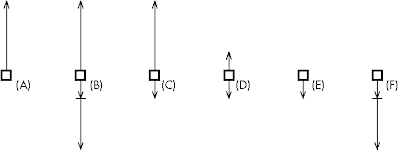[0.6 participation points.] Which free-body diagram represents a passenger (upside-down) at the top of a vertical loop, traveling fast enough that there is still contact with the seat?

Sections 0906, 0907
(A) : 1 student
(B) : 10 students
(C) : 10 students
(D) : 9 students
(E) : 1 student
(F) : 8 students

At the top of the circular arc, the net force points inwards (downwards), which is comprised of both the downwards normal force of the seat on the passenger, and the weight force of the Earth on the passenger.

[0.6 participation points.] Which free-body diagram represents a passenger (upside-down) at the top of a vertical loop, traveling just fast enough that there is barely contact with the seat?

Sections 0906, 0907
(A) : 0 students
(B) : 0 students
(C) : 2 students
(D) : 1 student
(E) : 35 student
(F) : 1 student

At the top of the circular arc, the net force points inwards (downwards), which is comprised only the downwards weight force of the Earth on the passenger.

## 20071010

### Uniform circular motion: wall of deathRhett "Rotten" Giordano, New Orleans Superdome Bike Expo
http://www.650motorcycles.com/83expoGrinder.jpg

Demonstration of how the (upwards) static friction force prevents the bike from sliding down the "Wall of Death," while the (inwards pointing) normal force provides the net force required for uniform circular motion.

## 20071009

### Uniform circular motion: centripetal net force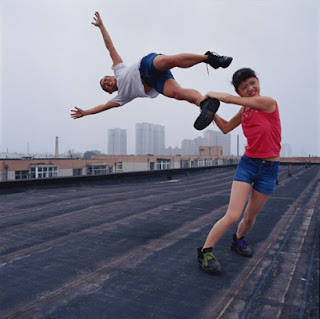Li Wei, liweiart.com
047-01, "Life in the high I" (detail)
Beijing July 1, 2004

Demonstration of the inward (centripetal) net force required for uniform circular motion.

## 20071008

### Astronomy clicker question: jovian weather patterns

Astronomy 10, Fall Semester 2007
Cuesta College, San Luis Obispo, CA

Astronomy 10 learning goal Q6.1

Students were asked the following clicker question (Classroom Performance System, einstruction.com) at the end of their learning cycle:

[0.3 points.] Which one of the following choices best explains why Saturn's belt-zones and cyclonic spot cloud features are less prominent than Jupiter's?
(A) Saturn supplies less tidal heating than Jupiter.
(B) Saturn retains much less heat than Jupiter.
(C) Saturn is farther from the Sun than Jupiter.
(D) Saturn has much less liquid metallic hydrogen than Jupiter.
(E) Saturn's rings are much more prominent than Jupiter's.

Correct answer: not revealed yet (see discussion).

Initial responses below:

Student responses
Section 1073
(A) : 6 students
(B) : 12 students
(C) : 3 students
(D) : 10 students
(E) : 2 students

The energy source of jovian planet belt-zone and cyclone weather patterns is core heat. A cooler core would result in less prominent weather patterns. The same question was asked again, with no explanation from the instructor.

[0.3 points.] Which one of the following choices best explains why Saturn's belt-zones and cyclonic spot cloud features are less prominent than Jupiter's?
(A) Saturn supplies less tidal heating than Jupiter.
(B) Saturn retains much less heat than Jupiter.
(C) Saturn is farther from the Sun than Jupiter.
(D) Saturn has much less liquid metallic hydrogen than Jupiter.
(E) Saturn's rings are much more prominent than Jupiter's.

Student responses
Section 1073
(A) : 6 students
(B) : 26 students
(C) : 0 students
(D) : 1 student
(E) : 0 students

## 20071005

### Erasing slate: study the night sky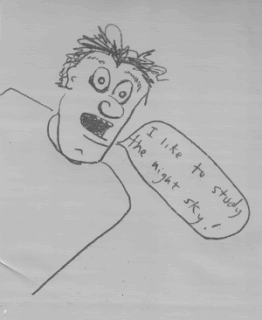"I like to study the night sky!" by Anonymous
Fall Semester 2007
Cuesta College, San Luis Obispo, CA

Latest scribbling on the lift-and-erase slate in the hallway, outside the office door.

## 20071003

### Physics quiz question: average speed versus average velocity

Physics 5A Quiz 3, Fall Semester 2007
Cuesta College, San Luis Obispo, CA

Cf. Giambattista/Richardson/Richardson, Physics, 1/e, Problem 3.26

[Version 1]

[3.0 points.] A car travels west at 80 km/h for 1.0 h. It then travels north at 45 km/h for 1.0 h. Which quantity has a larger magnitude for the car during this trip?
(A) The average speed.
(B) The average velocity.
(C) (Both average speed and average velocity have the same magnitude.)
(D) (Not enough information is given to determine this.)

The average speed is the distance traveled divided by the elapsed time, which is (80 km + 45 km)/2.0 h = 63 km/h. The average velocity is the displacement (straight-line distance from start to finish) divided by the elapsed time, which is sqrt((80 km)^2 + (45 km)^2)/2.0 h = 46 km/h.

Student responses
Sections 0906, 0907
(A) : 5 students
(B) : 10 students
(C) : 6 students
(D) : 0 students

[Version 2]

[3.0 points.] A car travels west at 80 km/h for 1.0 h. It then travels north at 45 km/h for 1.0 h. Which quantity has a smaller magnitude for the car during this trip?
(A) The average speed.
(B) The average velocity.
(C) (Both average speed and average velocity have the same magnitude.)
(D) (Not enough information is given to determine this.)

Student responses
Sections 0906, 0907
(A) : 7 students
(B) : 6 students
(C) : 7 students
(D) : 0 students

## 20071002

### Physics quiz question: friction-slowed book

Physics 205A (formerly Physics 5A) Quiz 3, fall semester 2007
Cuesta College, San Luis Obispo, CA

Cf. Giambattista/Richardson/Richardson, Physics, 1/e, Problems 4.26, 4.51

A Physics 205A student pushes an 2.00 kg book across the top of a horizontal table. The coefficient of kinetic friction is 0.3. After it is released, the book slides across the table, and because of friction, slows down with an acceleration of magnitude:
(A) 0.6 m/s2.
(B) 1 m/s2.
(C) 3 m/s2.
(D) 6 m/s2.

Correct answer (highlight to unhide): (C)

The book has two vertical forces acting on it:
Weight force of Earth on book (downwards, magnitude w = m·g = 19.6 N).
Normal force of floor on book (upwards, magnitude N = 19.6 N).
Because the book is stationary in the vertical direction, these two forces are equal in magnitude and opposite in direction, due to Newton's first law.

The book has only one horizontal force acting on it (as it moves to the right):
Kinetic friction force of floor on book (to the left).
The book is already unstuck and sliding (presumably to the right) after it being released, such that the only horizontal force acting on it is the (constant) kinetic friction force, directed to the left:

fk = µk·m·g = (0.3)(2.00 kg)(9.80 N/kg) = 6 N,

and from Newton's second law, the kinetic friction force (directed to the left) is the sole horizontal force that contributes to the (non-zero) horizontal net force (directed to the left), such that we can solve for the magnitude of the the horizontal acceleration:

ΣFx = –6 N,

m·ax = –6 N,

ax = (–6 N)/m = (–6 N)/2.00 kg) = –3 N/kg = –3 m/s2,

which has a magnitude of 3 m/s2 directed to the left.

(Response (A) is µk·m; response (B) is µk·g/m; and response (D) is the magnitude of the kinetic friction force fk = µk·m·g.)

Student responses
Sections 0906, 0907
(A) : 13 students
(B) : 2 students
(C) : 7 students
(D) : 19 students

Success level: 18%
Discrimination index (Aubrecht & Aubrecht, 1983): 0.50

## 20071001

### Astronomy quiz question: radioactive dating

Astronomy 10 Quiz 4, Fall Semester 2007
Cuesta College, San Luis Obispo, CA

Astronomy 10 learning goal Q4.2

[Version 1]

[3.0 points.] Consider three samples (X)-(Z), with differing amounts of unstable isotopes, embedded gaseous decay products, and inert material (which is not involved in the radioactive decay process), schematically shown below.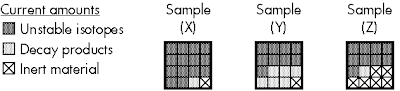Which one of the following choices best corresponds to the order of samples from youngest to oldest, as determined by radioactive dating?
(A) Youngest: X, Y, Z: oldest.
(B) Youngest: X, Z, Y: oldest.
(C) Youngest: Y, Z, X: oldest.
(D) Youngest: Y, X, Z: oldest.
(E) Youngest: Z, X, Y: oldest.

Student responses
Section 0135
(A) : 8 students
(B) : 16 students
(C) : 3 students
(D) : 2 students
(E) : 6 students

[Version 2]

[3.0 points.] Consider three samples (X)-(Z), with differing amounts of unstable isotopes, embedded gaseous decay products, and inert material (which is not involved in the radioactive decay process), schematically shown below.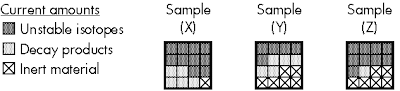Which one of the following choices best corresponds to the order of samples from youngest to oldest, as determined by radioactive dating?
(A) Youngest: X, Y, Z: oldest.
(B) Youngest: X, Z, Y: oldest.
(C) Youngest: Y, Z, X: oldest.
(D) Youngest: Y, X, Z: oldest.
(E) Youngest: Z, X, Y: oldest.